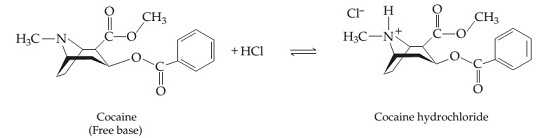# Problem: The "free-base" form of cocaine (C17H21NO4) and its protonated hydrochloride form (C17H22ClNO4) are shown below; the free-base form can be converted to the hydrochloride form with one equivalent of HCl. For clarity, not all the carbon and hydrogen atoms are shown; each vertex represents a carbon atom with the appropriate number of hydrogen atoms so that each carbon makes four bonds to other atoms.The free-base form of cocaine has a solubility of 1.00 g in 6.70 mL ethanol (CH3CH2OH). Calculate the molarity of a saturated solution of the free-base form of cocaine in ethanol.

###### FREE Expert Solution

We’re being asked to calculate the molarity of a saturated solution of the free-base form of cocaine in ethanol given its solubility.

Recall that molarity is the ratio of the moles of solute and the volume of solution (in liters). In other words:

We’re given the solubility of free-base cocaine in ethanol.  Recall that Solubility is the maximum amount of solute that can dissolve in a solvent.

• This means that only 1.00 g of free-base cocaine will dissolve in 6.70 mL ethanol (CH3CH2OH)
• A saturated solution contains the maximum amount of solute that can be dissolved in a solvent at a particular temperature.

The steps we need to do for this solution are:

Step 1: Calculate the moles of free-base cocaine (C17H21NO4

Step 2: Calculate the volume of the solution (in L).

Step 3: Calculate the molarity of the saturated solution.

93% (336 ratings)###### Problem Details

The "free-base" form of cocaine (C17H21NO4) and its protonated hydrochloride form (C17H22ClNO4) are shown below; the free-base form can be converted to the hydrochloride form with one equivalent of HCl. For clarity, not all the carbon and hydrogen atoms are shown; each vertex represents a carbon atom with the appropriate number of hydrogen atoms so that each carbon makes four bonds to other atoms.The free-base form of cocaine has a solubility of 1.00 g in 6.70 mL ethanol (CH3CH2OH). Calculate the molarity of a saturated solution of the free-base form of cocaine in ethanol.